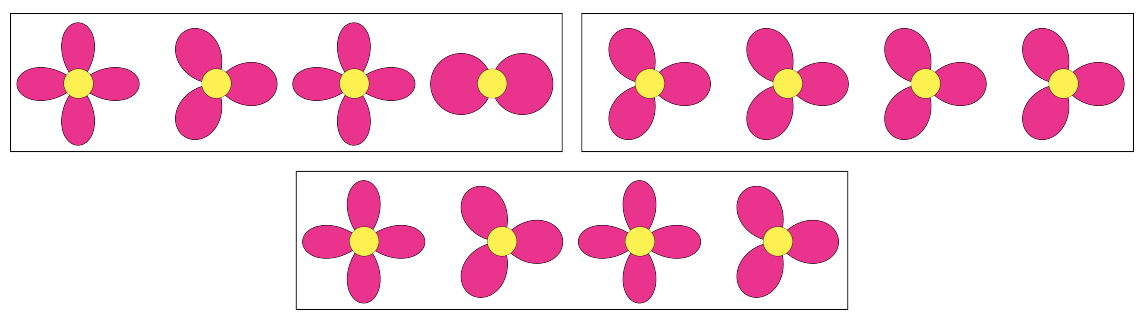시간 제한 메모리 제한 제출 정답 맞은 사람 정답 비율
1 초 512 MB 0 0 0 0.000%

## 문제

Gwen loves most numbers. In fact, she loves every number that is not a multiple of $n$ (she really hates the number $n$). For her friends' birthdays this year, Gwen has decided to draw each of them a sequence of $n-1$ flowers. Each of the flowers will contain between $1$ and $n-1$ flower petals (inclusive). Because of her hatred of multiples of $n$, the total number of petals in any non-empty contiguous subsequence of flowers cannot be a multiple of $n$. For example, if $n = 5$, then the top two paintings are valid, while the bottom painting is not valid since the second, third and fourth flowers have a total of $10$ petals. (The top two images are Sample Input $3$ and $4$.)Gwen wants her paintings to be unique, so no two paintings will have the same sequence of flowers.  To keep track of this, Gwen recorded each painting as a sequence of $n-1$ numbers specifying the number of petals in each flower from left to right.  She has written down all valid sequences of length $n-1$ in lexicographical order. A sequence $a_1,a_2,\dots, a_{n-1}$ is lexicographically smaller than $b_1, b_2, \dots, b_{n-1}$ if there exists an index $k$ such that $a_i = b_i$ for $i < k$ and $a_k < b_k$.

What is the $k$th sequence on Gwen's list?

## 입력

The input consists of a single line containing two integers $n$ ($2 \leq n \leq 1\,000$), which is Gwen's hated number, and $k$ ($1 \leq k \leq 10^{18}$), which is the index of the valid sequence in question if all valid sequences were ordered lexicographically. It is guaranteed that there exist at least $k$ valid sequences for this value of $n$.

## 출력

Display the $k$th sequence on Gwen's list.

## 예제 입력 1

4 3


## 예제 출력 1

2 1 2


## 예제 입력 2

2 1


## 예제 출력 2

1


## 예제 입력 3

5 22


## 예제 출력 3

4 3 4 2


## 예제 입력 4

5 16


## 예제 출력 4

3 3 3 3